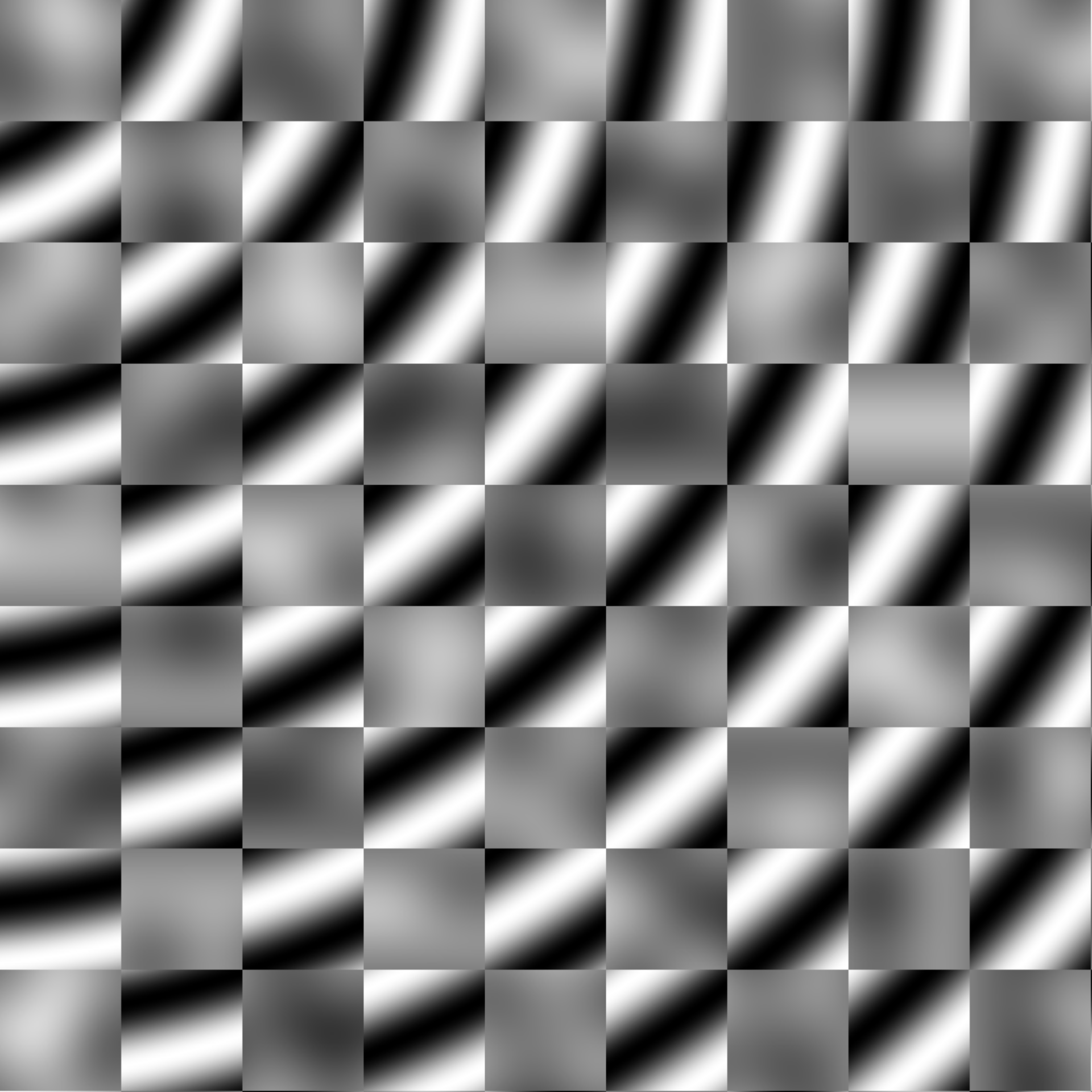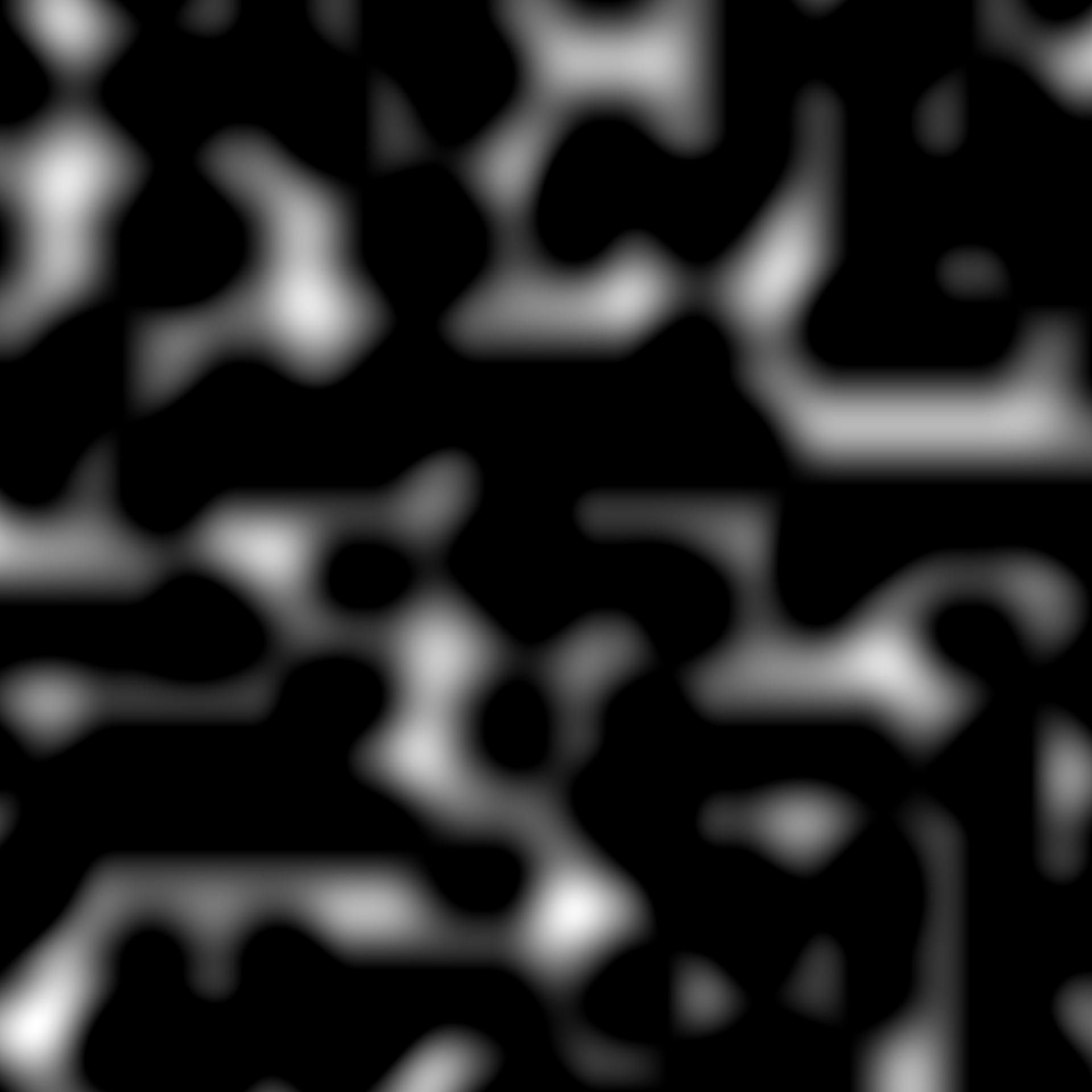Most modifications of values in a long_grid are quite simple due to the wealth of vectorised functions avaliable in R. ambient provides a little selection of handy functions to compliment these

blend(x, y, mask)

normalise(x, from = range(x), to = c(0, 1))

normalize(x, from = range(x), to = c(0, 1))

cap(x, lower = 0, upper = 1)

## Arguments

x, y Values to modify A vector of the same length as x and y. Assumed to be between 0 and 1 (values outside of this range is capped). The closer to 1 the more of x will be used and the closer to 0 the more of y will be used The range of x to use for normalisation The output domain to normalise to The lower and upper bounds to cap to

## Examples

grid <- long_grid(seq(1, 10, length.out = 1000), seq(1, 10, length.out = 1000))
grid$chess <- gen_checkerboard(grid$x, grid$y) grid$noise <- gen_perlin(grid$x, grid$y)
grid$ripple <- gen_waves(grid$x, grid$y) # Blend two values based on a third grid$mix <- blend(grid$noise, grid$ripple, grid\$chess)
plot(grid, mix)# Cap values between 0 and 1
plot(grid, cap(noise))